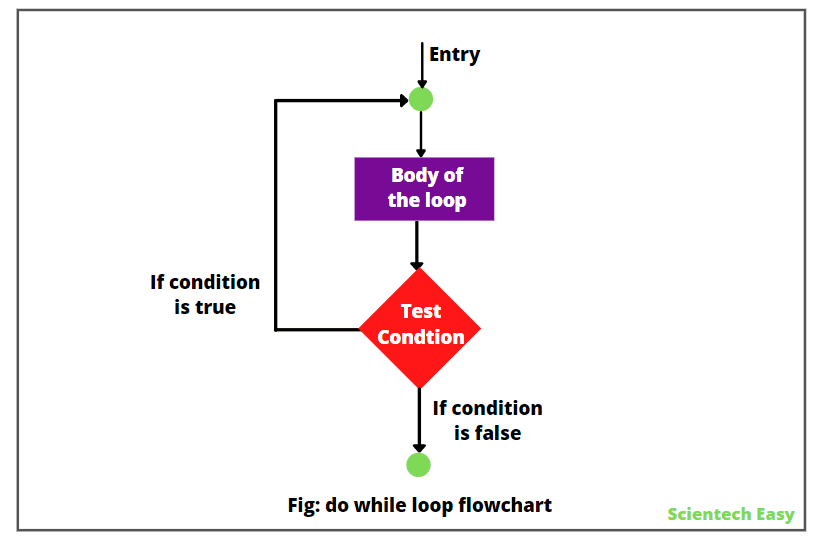# Do While Loop in Java | Example Program

A do-while loop in Java is a variant form of while loop. It is the same as a while loop, except that it executes the body of the loop first and then evaluates the loop continuation condition.

In the while loop statement, the test condition evaluates first before the loop body executes. If the condition is initially false, the body of loop will not execute at all.

However, sometimes, it might need to execute the body of a loop at least once before the test performs. We can handle such situations with the help of do-while loop in Java.

The do-while loop always executes its body at least once before the test evaluates because its test conditional expression is at the bottom of the loop.

## Syntax of do-while loop

The general syntax for using do-while loop in Java is as:

```  Initialization;
do {
// Loop body;
Statement(s);
Increment/decrement;
} while (test conditional expression);
```

## Flowchart Diagram of Do While Loop Statement

The execution flowchart for the do-while loop in Java has shown in the below figure.From the do-while loop flowchart, it is clear that the loop body executes first, and then the loop conditional expression evaluates to determine whether to continue or terminate the loop.

If the evaluation is true, the loop body executes again. This process continues until the test condition is true.

When the specified condition is false, the loop ends. The control of execution comes out of the do-while loop and goes to the next statement that appears immediately after the while statement.

Since the specified condition testes at the bottom of the loop, the do…while loop provides an exit-controlled loop. The test condition must be boolean expression.

We do not need to use curly braces if there is only one statement inside the do…while loop.

Consider the following example.

``` . . . . .
. . . . .
int i = 1, sum = 0;
do {
sum = sum + i;
i = i + 2;
}
while(sum < 40 || i < 10);
. . . . .
. . . . .
```

In this example, the body of loop will execute until one of the two relations is true.

## Example Program based on do while loop in Java

1. Let’s take a simple example program where we will display numbers from 1 to 6.

Program code 1:

```package javaProgram;
public class Test {
public static void main(String[] args)
{
int x;
x = 1; // starting number is 1.
do {
System.out.println(x); // print x value.
x++; // increment x value by 1.
} while(x <= 6); // This statement will execute as long as x <= 6.
}
}```
```Output:
1
2
3
4
5
6
```

a. In this example, the value of x is initially set to 1. Therefore, it is displayed 1.

b. Now, x++ statement will increment the value of x by 1, hence the value of x becomes 2. The conditional boolean expression x<=10 is tested.

c. Since this condition is true, the flow of execution will go back and the value of x, i.e. 2 will be displayed on the console.

d. Then the x value once again incremented and becomes 3. Since 3 is also less than 10, the flow once again goes back and displays x value.

e. In this way, this process continues until the value of x is less than or equal to 6.

f. As the value of x is greater than 6, the condition will be false and the loop will be terminated.

2. Let’s another example program based on do while statement where we will display the multiplication table.

Program code 2:

```package javaProgram;
public class Test {
public static void main(String[] args)
{
int row, column;
System.out.println("Multiplication Table \n");
row = 1; // Initialization.
do {
column = 1;
do{
int x = row * column;
System.out.printf("%4d", + x);
column = column + 1;
}while(column <= 5);
System.out.println("\n");
row = row + 1;
} while(row <= 5);
}
}```
```Output:
Multiplication Table:
1   2   3   4   5
2   4   6   8  10
3   6   9  12  15
4   8  12  16  20
5  10  15  20  25

```

In this example program, do-while loop is in nested form and produces the following output.

Program code 3:

```package javaProgram;
import java.util.Scanner;
public class Test {
public static void main(String[] args)
{
int num = 0, sum = 0;

// Create an object of Scanner class to take input.
Scanner sc = new Scanner(System.in);

// Continue reading data until the input is 0.
do {
System.out.println("Enter an integer number");
num = sc.nextInt();
sum = sum + num;
} while(num != 0);
System.out.println("Sum of numbers: " +sum);
}
}```
```Output:
Enter an integer number
20
Enter an integer number
10
Enter an integer number
5
Enter an integer number
40
Enter an integer number
0
Sum of numbers: 75
```

## Difference between while loop and do-while loop

The difference between while loop and do-while loop in Java is as follows:

1. In the case of while loop, first test condition is verified and then executes the statements inside the while block.

In the case of do-while loop, first statements inside do block are executed and then the test condition is verified.

2. If the test condition is false for first time, then block of statement executed zero times.

If the test condition is false for the first time, then the block of statement executed at least once time.

3. In the while syntax, while statement is at the top.

In the do-while syntax, while statement is at the bottom.

Hope that this tutorial has covered almost all the important points related to do while loop in Java with example programs. I hope that you will have understood this simple topic.

In the next tutorial, we will learn for loop in Java with example programs.